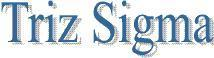Home Business Personal Social Blogs
Reliability
Introduction
A dimension of the quality of a products design is reliability. Reliability refers to the probability that the product will be functional when used. For example, reliability of a product being 95% means that 95 out of 100 products produced will perform as intended for a stated period of time, under specified operating conditions.

• Each part of a product is designed for a given level of component reliability
• Component reliability is defined as the probability that a part will not fail in a given time period or number of trials under ordinary conditions of use
• Components
3 common measures of component reliability are:

 Reliability (CR) CR = 1 - FR Failure Rates (FR and FRn) FR = (Number of failure /Number tested),FRn = (Number of failure/Unit hours of operations) Mean Time Between Failures (MTBF) MTBF=(Unit hours of operations/Number of failure)=(1/FRn)

Failure rate is a measure used to ascertain reliability of a product or a process. For products that must be replaced because they are dysfunctional, a relevant measure of interest is the mean time to failure (MTTF), and for products that may be repaired and put to service again, a relevant measure of interest is mean time between failures (MTBF). In order to calculate MTTF and MTBF, ideally, we would consider a large number of products to be operated and tested until failure and record the time of the failure for each. The information about MTTF and MTBF helps ascertain reliability. However, it is time consuming and costly to collect enough data to build a probability distribution and cumulative distribution curve of time to failure or time between failures. One way we can deal with this problem is by analyzing a smaller set of data and identifying a distribution that approximates the distribution of time to failure, such as the Exponential, Weibull, or Gamma distributions. Once we have identified a distribution, we can easily calculate failure rate, MTTF, etc.

The combined reliability of all the components in a product forms the basis for system reliability (SR) When n independent critical components are combined into a product, the SR is determined by:

SR = CR1 x CR2 x CR3 x  x CRn

Consider a product with 50 identical critical components:

• If each components CR = 99.5%, then SR = 77.8%
• If each components CR = 98.0%, then SR = 36.4%
• If each components CR = 90.0%, then SR = 0.5%
Example
If the machine could be redesigned to allow redundancy for the component that presently has a reliability of .90, what would be the new system reliability of the machine?First, compute the CR for the redundant parts.

CR = Probability of primary component working + [(Probability of backup component working) x (Probability of needing backup component)]

= .90 + [(.90) x (.10)] = .90 + .09 = .99

Now, compute the system reliability.

SR = (.96) (.99) (.98) = .931

 Copyright 2008, trizsigma.com. All rights reserved. Designed and Hosted by Mirage Solutions